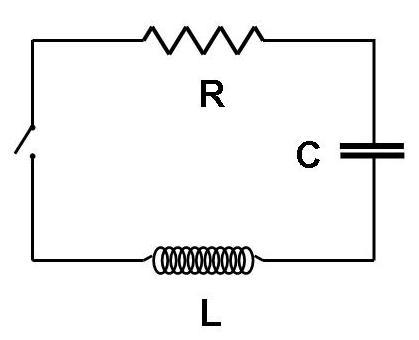LRC Circuit

Homework Statementa. What is the frequency of the current oscillation of the circuit as it is shown?

b. What is the frequency of the oscillations in electrical potential difference, V, between the two ends of the resistor in the circuit as it is shown?

c. What is the charge on the capacitor after the current has oscillated back and forth once?

Homework Equations

$$\omega$$ = 1/(sqrt[LC]) --LaTeX's sqrt wasn't working

The Attempt at a Solution

I can easily find the freq of the current oscillations using the formula above, but I can't recall what the formula is for damped freq. Once I know that, I'm fairly certain I'll be able to solve the rest.

ehild
Homework Helper
Was the capacitor charged before closing the switch? Are you sure you wrote the problem correctly? What do current oscillation and potential difference oscillation mean?

ehild

Alright I found the formula for dampened oscillations. In case someone wants to know it's:
w' = w^2 - b^2

where b = R/(2L) and w = 1/sqrt[LC]

gneill
Mentor
Look up the Q-factor for an LRC circuit. How's it defined?